SSC (Marathi Semi-English) 10thMaharashtra State Board
Share

# Geometry Shaalaa.com Model Set 3 2019-2020 SSC (Marathi Semi-English) 10th Question Paper Solution

Geometry [Shaalaa.com Model Set 3]
Marks: 40Date: 2019-2020 March
Duration: 2h

1
1.A | MCQs
1.A.i

In ∆ABC and ∆DEF ∠B = ∠E, ∠F = ∠C and AB = 3DE then which of the statements regarding the two triangles is true ?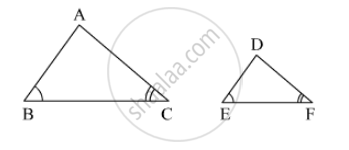The triangles are not congruent and not similar

The triangles are similar but not congruent.

The triangles are congruent and similar.

None of the statements above is true.

Concept: Similarity of Triangles
Chapter:  Similarity
1.A.ii

Four alternative answers for the following question is given. Choose the correct alternative.
If two circles are touching externally, how many common tangents of them can be drawn?

(A) One (B) Two (C) Three (D) Four
Concept: Tangent to a Circle
Chapter:  Circle
1.A.iii

Choose the correct alternative answer for the following question.

When we see at a higher level , from the horizontal line,angle formed is ....... .
(A) angle of elevation.
(B) angle of depression.
(C) 0
(D) straight angle.
Concept: Application of Trigonometry
Chapter:  Trigonometry
1.A.iv

Select the correct alternative for the following question.

The maximum number of tangents that can be drawn to a circle from a point out side it is .............. .

(A) 2 (B) 1 (C) one and only one (D) 0
Concept: Construction of Tangent to the Circle from the Point on the Circle
Chapter:  Geometric Constructions
1.B
1.B.i

Determine whether the following point is collinear.
A(–1, –1), B(0, 1), C(1, 3)

Concept: Slope of a Line
Chapter:  Co-ordinate Geometry
1.B.ii

Prove the following.
secθ (1 – sinθ) (secθ + tanθ) = 1

Concept: Application of Trigonometry
Chapter:  Trigonometry
1.B.iii

Base of a triangle is 9 and height is 5. Base of another triangle is 10 and height is 6. Find the ratio of areas of these triangles.

Concept: Similar Triangles
Chapter:  Similarity
1.B.iv

Find the total surface area of a cylinder if the radius of its base is 5 cm and height is 40 cm.

Concept: Introduction of Surface Areas and Volumes
Chapter:  Mensuration
2
2.A | Solve any 2 of the following
2.A.i

In the given figure, a toy made from a hemisphere, a cylinder and a cone is shown. Find the total area of the toy.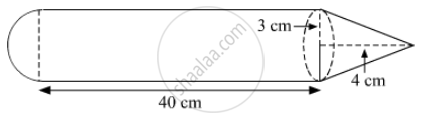Concept: Surface Area of a Combination of Solids
Chapter:  Mensuration
2.A.ii

In ∆MNP, NQ is a bisector of ∠N. If MN = 5, PN = 7 MQ = 2.5 then Find QP.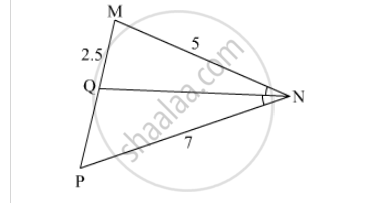Concept: Property of an Angle Bisector of a Triangle
Chapter:  Similarity
2.A.iii

In ∆PQR, point S is the midpoint of side QR. If PQ = 11, PR = 17, PS =13, find QR.

Concept: Pythagoras Theorem
Chapter:  Pythagoras Theorem
2.B | Solve any 4 of the following
2.B.i

Find the length a diagonal of a rectangle having sides 11 cm and 60 cm.

Concept: Apollonius Theorem
Chapter:  Pythagoras Theorem
2.B.ii

What is the distance between two parallel tangents of a circle having radius 4.5 cm ? Justify your answer.

Concept: Tangent to a Circle
Chapter:  Circle
2.B.iii

∆ABC ~ ∆LMN. In ∆ABC, AB = 5.5 cm, BC = 6 cm, CA = 4.5 cm. Construct ∆ABC and ∆LMN such that $\frac{BC}{MN} = \frac{5}{4} .$

Concept: Construction of Triangle If the Base, Angle Opposite to It and Either Median Altitude is Given
Chapter:  Geometric Constructions
2.B.iv

A boy standing at a distance of 48 meters from a building observes the top of the building and makes an angle of elevation of 30°. Find the height of the building.

Concept: Heights and Distances
Chapter:  Trigonometry
2.B.v

In the given figure, seg RS is a diameter of the circle with centre O. Point T lies in the exterior of the circle. Prove that ∠ RTS is an acute angle.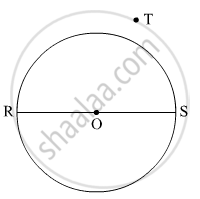Concept: Inscribed Angle
Chapter:  Circle
3
3.A | Solve any 1 of the following
3.A.i

In the given figure, ∠DFE = 90°, FG ⊥ ED, If GD = 8, FG = 12, find (1) EG (2) FD and (3) EF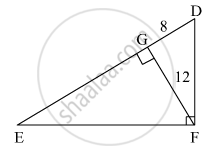Concept: Pythagoras Theorem
Chapter:  Pythagoras Theorem
3.A.ii

In the given figure, ▢PQRS is cyclic. side PQ ≅ side RQ. ∠ PSR = 110°, Find–
(1) measure of ∠ PQR
(2) m(arc PQR)
(3) m(arc QR)
(4) measure of ∠ PRQ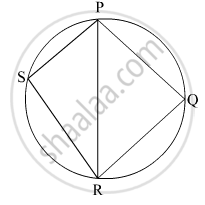Concept: Property of Sum of Measures of Arcs
Chapter:  Circle
3.B | Solve any 2 of the following
3.B.i

Find the coordinates of the circumcentre of a triangle whose vertices are (–3,1), (0,–2) and (1,3).

Concept: Concepts of Coordinate Geometry
Chapter:  Co-ordinate Geometry
3.B.ii

In the given figure, seg PA, seg QB, seg RC and seg SD are perpendicular to line AD. AB = 60, BC = 70, CD = 80, PS = 280 then Find PQ, QR and RS.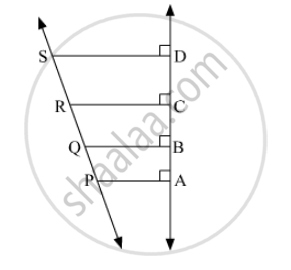Concept: Similarity of Triangles
Chapter:  Similarity
3.B.iii

If 5 secθ – 12 cosecθ = 0, find the values of secθ, cosθ and sinθ.

Concept: Application of Trigonometry
Chapter:  Trigonometry
3.B.iv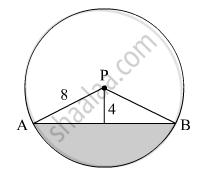In the given figure, seg AB is a chord of a circle with centre P. If PA = 8 cm and distance of chord AB from the centre P is 4 cm, find the area of the shaded portion. ( $\pi$ = 3.14, $\sqrt{3}$= 1.73 )

Concept: Surface Area of a Combination of Solids
Chapter:  Mensuration
4 | Solve any 2 of the following
4.A

Construct a triangle of sides 4 cm, 5cm and 6cm and then a triangle similar to it whose sides are 2/3 of the corresponding sides of the first triangle. Give the justification of the construction.

Concept: Division of a Line Segment
Chapter:  Geometric Constructions
4.B

Two concentric circles are of radii 5 cm and 3 cm. Find the length of the chord of the larger circle which touches the smaller circle

Concept: Number of Tangents from a Point on a Circle
Chapter:  Circle

The length of a tangent from a point A at distance 5 cm from the centre of the circle is 4 cm. Find the radius of the circle.

Concept: Number of Tangents from a Point on a Circle
Chapter:  Circle
4.C

A chord PQ of a circle with radius 15 cm subtends an angle of 60° with the centre of the circle. Find the area of the minor as well as the major segment. ( $\pi$ = 3.14,  $\sqrt{3}$ = 1.73)

Concept: Areas of Sector and Segment of a Circle
Chapter:  Mensuration

In the given figure, A is the centre of the circle. $\angle$ABC = 45° and AC = 7 $\sqrt{2}$cm. Find the area of segment BXC.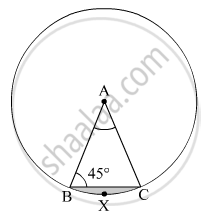Concept: Areas of Sector and Segment of a Circle
Chapter:  Mensuration
5 | Solve any 1 of the following
5.A

Find the lengths of the medians of a triangle whose vertices are A(–1, 1), B(5, –3) and C(3, 5).

Concept: Centroid Formula
Chapter:  Co-ordinate Geometry
5.B

In the given figure 1.66, seg PQ || seg DE, A(∆PQF) = 20 units, PF = 2 DP, then Find A(◻DPQE) by completing the following activity.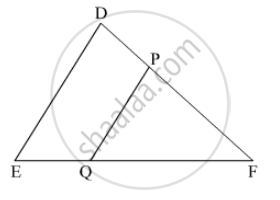Concept: Areas of Two Similar Triangles
Chapter:  Similarity

#### Request Question Paper

If you dont find a question paper, kindly write to us

View All Requests

#### Submit Question Paper

Help us maintain new question papers on Shaalaa.com, so we can continue to help students

only jpg, png and pdf files

## Maharashtra State Board previous year question papers 10th Geometry with solutions 2019 - 2020

Maharashtra State Board 10th Geometry question paper solution is key to score more marks in final exams. Students who have used our past year paper solution have significantly improved in speed and boosted their confidence to solve any question in the examination. Our Maharashtra State Board 10th Geometry question paper 2020 serve as a catalyst to prepare for your Geometry board examination.
Previous year Question paper for Maharashtra State Board 10th Geometry-2020 is solved by experts. Solved question papers gives you the chance to check yourself after your mock test.
By referring the question paper Solutions for Geometry, you can scale your preparation level and work on your weak areas. It will also help the candidates in developing the time-management skills. Practice makes perfect, and there is no better way to practice than to attempt previous year question paper solutions of Maharashtra State Board 10th.

How Maharashtra State Board 10th Question Paper solutions Help Students ?
• Question paper solutions for Geometry will helps students to prepare for exam.
• Question paper with answer will boost students confidence in exam time and also give you an idea About the important questions and topics to be prepared for the board exam.
• For finding solution of question papers no need to refer so multiple sources like textbook or guides.
S